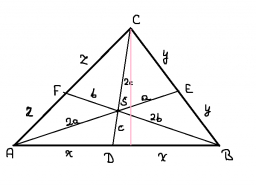# The number 5

The number of hours per week the television is turned on is determined for each family in a sample. The mean of the data is 35 hours, and the median is 31.2 hours. Twenty-four families in the sample turned on the television for 20 hours or less for the week. The 6th percentile of the data is 20 hours.

Question: Approximately how many families are in the sample? Round your answer to the nearest integer.

n =  333

### Step-by-step explanation:Did you find an error or inaccuracy? Feel free to write us. Thank you!

Tips for related online calculators
Looking for help with calculating arithmetic mean?
Looking for a statistical calculator?
Looking for a standard deviation calculator?